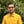Trusted answers to developer questions
Trusted Answers to Developer Questions

Related Tags

scala
math
cosine
communitycreator

# What is math.cos() in Scala?Harris Amjad

Grokking Modern System Design Interview for Engineers & Managers

Ace your System Design Interview and take your career to the next level. Learn to handle the design of applications like Netflix, Quora, Facebook, Uber, and many more in a 45-min interview. Learn the RESHADED framework for architecting web-scale applications by determining requirements, constraints, and assumptions before diving into a step-by-step design process.

The cos() function in Scala returns the cosine of a number. To be more specific, it returns the cosine of a number in radians.

Figure 1 below shows the mathematical representation of the cos() function.

Figure 1: Mathematical representation of cosine function

The scala.math._ header file is required for this function.

This cos() function only works for right-angled triangles.

### Syntax

Double cos(Double number)


### Parameter

This function requires a number that represents an angle in radians as a parameter.

To convert degrees to radians, use the formula below.

radians = degrees * ( Pi / 180.0 )


### Return value

cos() returns the cosine of a number in radians that is sent as a parameter.

If the parameter value is NaNNot a Number or positive infinity or negative infinity, it returns NaN.

### Code

import scala.math._object Main extends App {    //positive number in radians    println(s"The value of cos(2.3) = ${cos(2.3)}"); // negative number in radians println(s"The value of cos(-2.3) =${cos(-2.3)}");     //converting the degrees angle into radians and then applying cos()    // degrees = 45    // PI = 3.14159265    // result first converts degrees to radians then apply cos    println(s"The value of cos(45) = ${cos(45 * (Pi / 180))}"); //error outputs println(s"The value of cos(Double.PositiveInfinity) =${cos(Double.PositiveInfinity)}");    println(s"The value of cos(Double.NegativeInfinity) = ${cos(Double.NegativeInfinity)}"); println(s"The value of cos(Double.NaN) =${cos(Double.NaN)}");}

RELATED TAGS

scala
math
cosine
communitycreator

CONTRIBUTORHarris Amjad

Grokking Modern System Design Interview for Engineers & Managers

Ace your System Design Interview and take your career to the next level. Learn to handle the design of applications like Netflix, Quora, Facebook, Uber, and many more in a 45-min interview. Learn the RESHADED framework for architecting web-scale applications by determining requirements, constraints, and assumptions before diving into a step-by-step design process.

Keep Exploring

Learn in-demand tech skills in half the time